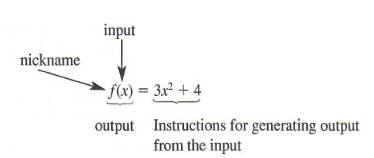Home | About | Contact | Disclaimer | Help

Algebra

Equations

Inequalities

Graphs

Numbers

Calculus

Matrices

Tutorials

### Help: Miscellaneous functions

Here is a complete list of miscellaneous functions accepted by QuickMath. The tables show the usual form in which the functions appear in textbooks, along with the form accepted by QuickMath. In most cases, the QuickMath version is identical to the textbook version.

If there is a function missing which you would like see added to those supported by QuickMath, just send your suggestion to contact form.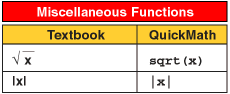# Functions overview

The concept of a function is central to mathematics. As we shall see, functions are used to model special relationships between quantities and an understanding of how functions work and different ways to represent functions will be invaluable for solving a variety of problems. In particular, the ability to analyze a function graphically is a significant benefit when the function is modeling a relationship between two quantities that is nonlinear, so that rate of change is not constant.

## Functions from Numerical and Algebraic View

OBJECTIVES

1. Define the Concept of Function
2. Describe Functions Numerically and Algebraically
3. Use Functional Notation
2.1 Functions from a Numerical and Algebraic View

Consider the following statements.

1. "Income tax is a function of how much you earn."
2. "My grade on an exam is a function of how much time I study."
3. "TV ad price is a function of ratings."
We could easily replace the phrase "is a function of' with "depends on" in each statement. This kind of relationship between quantities, where one quantity depends on another quantity (or quantities), is a fundamental idea in mathematics. We use the term function to refer to any such relationship. In particular, we will investigate the idea of one quantity being a function of another quantity.

What Is a Function?
As just stated, in the most general case we think of a function as a relationship between quantities, where one quantity determines the other. It is useful, when we say "quantity B is a function of quantity A," to think of particular values of quantity A as inputs and corresponding values of quantity B as outputs, with some rule (or process) prescribing how any particular value of quantity A determines precisely one corresponding value of quantity B. More formally, we define a real-valued function of a real variable in three parts, as follows:

A function consists of:

1. A collection of real number inputs, called the domain of the function
2. A collection of corresponding real number outputs, called the range of the function
3. A rule of correspondence that describes how each particular input generates exactly one corresponding output

It is important to note here that each input for a given function generates only one corresponding output, but distinct inputs may actually generate the same output.The following table is adapted from the 2011 Form 1040 Federal Income Tax booklet. It describes federal income tax owed as a function of taxable income for a filer filing as single. It shows, in theory, how every taxable income level generates a corresponding tax liability.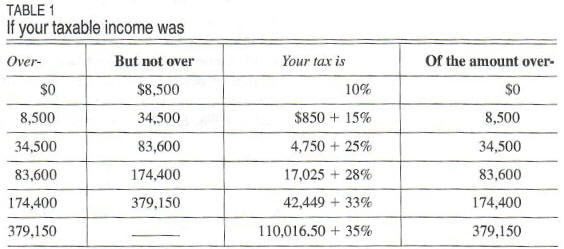The domain of the "income tax function" is al1 possible taxable incomes, represented by the real number interval (0, oo). The range is the collection of all possible corresponding tax amounts, which the interval (0, oo) also represents. A question of some merit is, "How do we describe functions?" In some cases, a verbal description of domain, range and rule of correspondence may indeed be pos sible (imagine doing this with the income tax function above), but it is typically not a practical approach. In the situation above, the table provided an adequate display of the rule of correspondence between taxable income and tax liability, and from the context we were able to deduce a domain and range. We now tum our attention to ways of describing functions.

Describing Functions Numerically
Table 2 has as its basis information from the periodical The New York Times. It lists the approximate U.S. oil imports from Mexico for 2001-2006.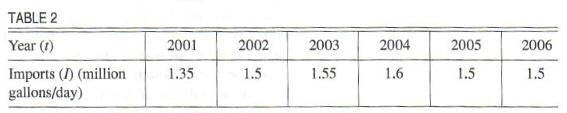This table describes oil imports,I, as a function of year, t. The domain is the set D = { 200 I, 2002, 2003, 2004, 2005, 2006} while the range is the set R = { l.35, 1.5, 1.55, 1.6)
The table gives the rule of correspondence between t and I. Notice, however, that the table does not describe t as a function of I, as the value I = 1.5 corresponds to t = 2002, t = 2005, and t = 2006. We say here that I is described numerically as a function of t, as the table gives specific values of I corresponding to specific values of t.
In the situation given above, since the description of I was as a function of t, we say that t is the independent variable and I is the corresponding dependent variable. The distinction between independent variables and dependent variables will become more important later.
Table 3 below gives the median age of the U.S. population for certain years, as recorded by the U.S. Bureau of the Census in Statistical Abstract of the United States, 1998. The table describes median age as a function of year.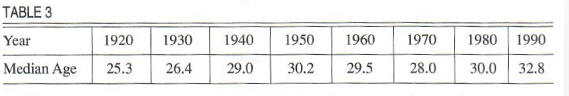We will use the notation M(y) (read "M of y") to denote the median age of the U.S. population in year y. Specifically, M( 1920) is the median age in 1920, M(1930) is the median age in 1930, and so on. Consequently, we write M(1920) = 25.3, M(1930) = 26.4, etc. We call this notation functional notation, and it is a useful shorthand for describing the relationship between inputs and outputs for a given function. In particular, mathematicians commonly use it when describing functions algebraically. We now turn our attention to that method of describing functions.

Describing Functions Algebraically
When we use an explicit formula or an equation to describe how inputs and outputs are related, we say that we are describing a function algebraically. For instance, consider the familiar formula for the area, A, of a circle of radius r: A = pi*r2. Considering r as the independent variable, this equation describes A as a function of r. For each particular radius, we have an explicit set of instructions for how to calculate the corresponding area of the circle with that radius, namely, "square the radius
and multiply the result by x" In this context, a natural domain and a corresponding range arise for the function in question. The domain is all possible radii r (so, all positive real numbers), and the range is the set of all corresponding areas, which is also the set of all positive real numbers. Using interval notation, the interval (0, oo) gives both the domain and the range.

The usefulness of functional notation becomes more apparent when working with functions described algebraically. For instance, consider the equation f(x) = 3x2 + 4. As before, when using this notation, x represents the input and the symbol f (x) represents the corresponding output. The letter f is nothing more than a "nick name" for the function described. The equation, taken as a whole, gives the rule of the function: